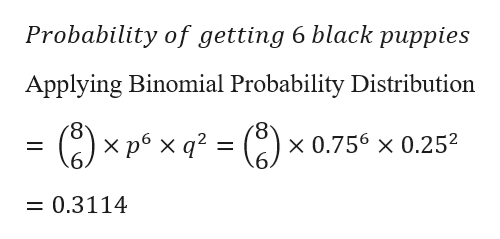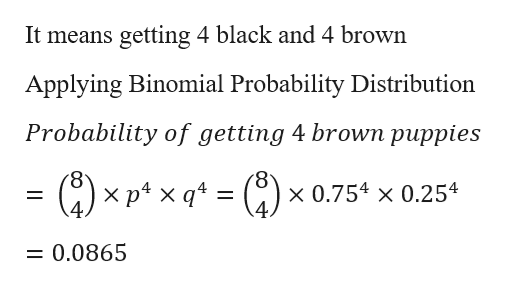# 3) Mendelian genetics predicts that in Labrador retrievers (a type of dog), 75% should have black color if both parents are heterozygous (the rest are brown). You mate two parents and get a litter of 8 puppies. Calculate the following:a) The probability of 6 black puppies.b) The probability of 4 brown puppies.c) The probability that 25% of the puppies will be brown.

Question
21 views

3) Mendelian genetics predicts that in Labrador retrievers (a type of dog), 75% should have black color if both parents are heterozygous (the rest are brown). You mate two parents and get a litter of 8 puppies. Calculate the following:

a) The probability of 6 black puppies.

b) The probability of 4 brown puppies.

c) The probability that 25% of the puppies will be brown.

check_circle

Step 1

Probability that the dog is of black colour = 0.75

Probability that the dog is of brown colour = 0.25

N = 8

Applying Binomial Probability Distribution

Step 2

a)
Probability of 6 black puppies
Black puppies p = 0.75
Brown puppies q = 0.25
Probability of getting 6 black puppieshelp_outlineImage TranscriptioncloseProbability of getting 6 black puppies Applying Binomial Probability Distribution x 0.756 x 0.252 X 0.3114 fullscreen
Step 3

b) Probability of getting 4 brown puppies

It ...help_outlineImage TranscriptioncloseIt means getting 4 black and 4 brown Applying Binomial Probability Distribution Probability of getting 4 brown puppies - C) x 0.754 x 0.254 X X 0.0865 fullscreen

### Want to see the full answer?

See Solution

#### Want to see this answer and more?

Solutions are written by subject experts who are available 24/7. Questions are typically answered within 1 hour.*

See Solution
*Response times may vary by subject and question.
Tagged in

### Other